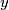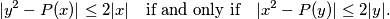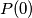IMO Shortlist 2014 problem A5

Kvaliteta:
Avg: 2,0
Težina:
Avg: 8,0

Consider all polynomials$P(x)$ with real coefficients that have the following property: for any two real numbers$x$ and$y$ one hasDetermine all possible values of$P(0)$.

(Belgium)

Izvor: https://www.imo-official.org/problems/IMO2014SL.pdf